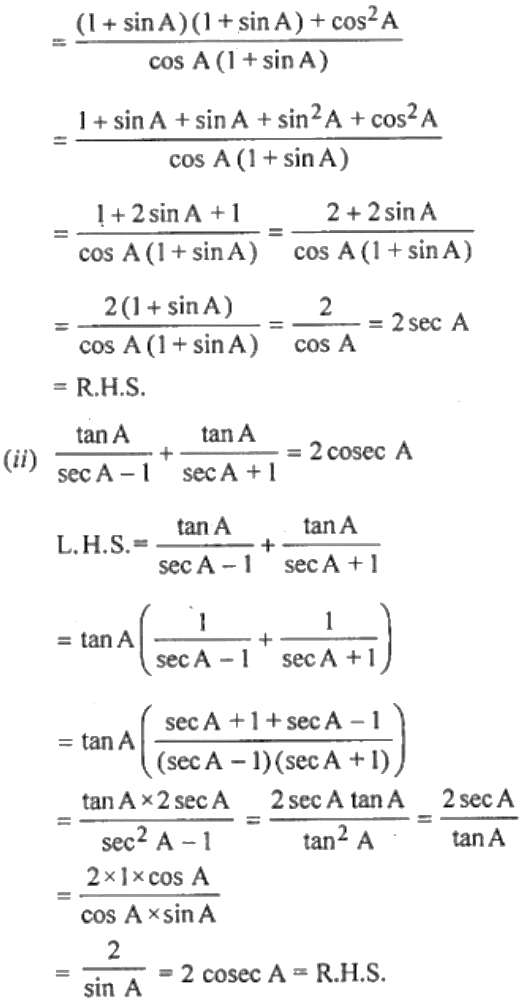Guru

# (i) (1 + sin A)/ cos A + cos A/ (1 + sin A) = 2 sec A (ii) tan A/ (sec A – 1) + tan A/ (sec A + 1) = 2cosec A

• 0

This is an important question taken from the Book Book- ML Aggarwal
Board- ICSE
Publication- Avichal
Chapter- Trigonometric Identities
Chapter number-18

This ques has been asked in previous year question paper of class 10 we

have prove. That

(i) (1 + sin A)/ cos A + cos A/ (1 + sin A) = 2 sec A (ii) tan A/ (sec A – 1) + tan A/ (sec A + 1) = 2cosec A

Class 10, chapter 18, ICSE, ques no 17

Share